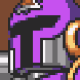This shader is a combination of the knowledge I gained from these two tutorials:

The environment is not by me and should thereby not be used in projects (unless you have the appropriate license for it). I simply used it for showcasing purposes.

Low Poly grass, stones and log by:

https://quaternius.com/packs/ultimatenature.html

If you have suggestions to e.g. add an underwater effects or improve the shader, feel free to open an issue on the repo.

``````shader_type spatial;
render_mode specular_phong, cull_disabled;

uniform float speed: hint_range(-1, 1) = 0.0;

uniform sampler2D noise1;
uniform sampler2D noise2;
uniform sampler2D normalmap: hint_normal;

uniform vec4 color : hint_color;
uniform vec4 deep_water: hint_color;

uniform float beer_law_factor = 2.0;
uniform float _distance = 0.0;

//foam var
uniform vec4 edge_color: hint_color;
uniform float edge_scale = 0.25;
uniform float near = 0.1;
uniform float far = 100f;

// wave var
uniform vec2 wave_strength = vec2(0.5, 0.25);
uniform vec2 wave_frequ = vec2(12.0, 12.0);
uniform vec2 time_factor = vec2(1.0, 2.0);

float waves(vec2 pos, float time) {
return (wave_strength.y * sin(pos.y * wave_frequ.y + time * time_factor.y)) + (wave_strength.x * sin(pos.x * wave_frequ.x + time * time_factor.x));
}

void vertex() {
VERTEX.y += waves(VERTEX.xy, TIME);
}

float rim(float depth) {
depth = 2f * depth - 1f;
return near * far / (far + depth * (near - far));
}

vec4 fragcoord, float beer_factor, float __distance, vec3 vertex) {

float scene_depth = depth;

scene_depth = scene_depth * 2.0 - 1.0;
scene_depth = projection_matrix / (scene_depth + projection_matrix);
scene_depth = scene_depth + vertex.z; // z is negative

// application of beers law
scene_depth = exp(-scene_depth * beer_factor);

float screen_depth = fragcoord.z;

float depth_fade = (scene_depth - screen_depth) / __distance;

}

void fragment() {
float time = TIME * speed;

vec3 n1 = texture(noise1, UV + time).rgb;
vec3 n2 = texture(noise2, UV - time * 0.2).rgb;

vec2 uv_movement = UV * 4f;
uv_movement += TIME * speed * 4f;

float sum = (n1.r + n2.r) - 1f;

float z_depth = rim(texture(DEPTH_TEXTURE, SCREEN_UV).x);
float z_pos = rim(FRAGCOORD.z);
float diff = z_depth - z_pos;

vec2 displacement = vec2(sum * 0.1);
diff += displacement.x * 70f;

vec4 col = mix(edge_color, gradientcolor, step(edge_scale, diff));

vec4 alpha = texture(SCREEN_TEXTURE, SCREEN_UV + displacement);

float fin = 0.0;
if (sum > 0.0 && sum < 0.4) fin = 0.1;
if (sum > 0.4 && sum < 0.8) fin = 0.0;
if (sum > 0.8) fin = 1f;

// konvertier fin in vec3 um
ALBEDO = vec3(fin) + mix(alpha.rgb, col.rgb, gradientcolor.a);

NORMALMAP = texture(normalmap, uv_movement).rgb;

ROUGHNESS = 0.1;
}``````
###### TagsThe shader code and all code snippets in this post are under MIT license and can be used freely. Images and videos, and assets depicted in those, do not fall under this license. For more info, see our License terms.

### Stylized fire (3D)Inline Feedbacks1 year ago

I’m using your shader in my game and I don’t know why but sometimes it “swallows” other shaders and/or MeshInstances: https://imgur.com/a/Bc8Ob0b (Notice how both the exclamation mark and the smoke disappear where the water is.)

Last edited 1 year ago by telmo1 year ago